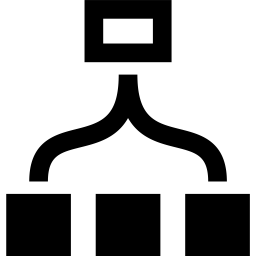# QN

A QN object contains set of "quantum numbers" (up to four of these; this number can be increased with a compile-time flag).

Quantum numbers have a name and an integer value. They can also optionally have a "mod factor" which makes them obey $Z_N$ addition rules rather than standard integer addition.

QN objects can be added and subtracted, and any missing quantum numbers are treated as having the value zero.

Similarly, QN objects can be compared, and any missing quantum numbers are treated as having the value zero.

## Synopsis

auto q1 = QN({"Sz",1});
auto q2 = QN({"Sz",2});

Print(q1 == q1); //prints "true"
Print(q1 == q2); //prints "false"

Print(q1+q2); //prints QN({"Sz",3})
Print(q1-q1); //prints QN({"Sz",0})
Print(q1-q2); //prints QN({"Sz",-1})

auto c = QN({"C",1});

Print(q1+c); //prints QN({"C",1},{"Sz",1})

auto qc0 = QN({"C",0},{"Sz",0});
auto c0 = QN({"C",0});

Print(qc0 == c0); //prints "true", since
//missing quantum numbers
//are treated as zero


When describing spin quantum numbers (total Sz, say), our convention is to work in units of spin 1/2. Thus a QN object made as QN("Sz=",+1) means Sz=+0.5 in physics units. The reason for this is that computers can perform integer arithmetic quickly and exactly. For particle quantum numbers (such as total boson or fermion number) integer values have their usual meaning.

Each quantum number in a QN object has a modulus which can take the following types of values:

• If the modulus is 1, the quantum number follows regular integer addition.
• If the modulus is $N>1$ , the quantum number obeys $Z_N$ addition.
• If the modulus is $-N \leq 1$ , the quantum number is fermionic. A fermionic quantum number follows the same addition rules as a bosonic one of modulus $|N|$ ; the minus sign on the modulus acts only as a "flag" indicating fermionic statistics. (As of version 3.0.0 of ITensor we do not use these fermionic flags for any purpose, but have implemented them for future experimental automatic fermionic features to be released.)

QN is defined in the header "itensor/qn.h".

## QN Class Methods

• QN()


Default constructor. Results in a QN with no explicit quantum numbers. In a context such as addition or comparison, missing quantum numbers are treated as having the value zero.

• QN(QNum v1)

QN(QNum v1, QNum v2)

QN(QNum v1, QNum v2, QNum v3)

QN(QNum v1, QNum v2, QNum v3, QNum v4)


Construct a QN by providing up to four quantum numbers. Quantum number (QNum) objects can be conveniently constructed by providing a braced list of the form {"Name",val} or {"Name",val,mod} where "Name" is the name of the quantum number (up to 7 characters), val is its value (an integer), and mod is the optional modulus described above.

Click to Show Example
  auto q = QN({"Sz",0},{"N",2});

auto v = QN({"P",1,3});

• QN(int val)


For convenience, a QN object can be constructed by just providing a single integer value. The single quantum number it contains will not have a name.

• .size() -> size_t

Return the number of quantum numbers (QNums) in this QN.

• .val(QName name) -> int

Retrive the value of the quantum number with the given name (a string). If the QN has no quantum number with this name, returns 0.

• .mod(QName name) -> int

Retrive the modulus of the quantum number with the given name (a string). If the QN has no quantum number with this name, returns 0.

• .num(QName name) -> QNum

Retrieve a quantum number by providing its name (a QName == SmallString).

• .addNum(QNum num)

Add a quantum number (QNum) to this QN. If the QN already has a quantum number with the same name, results in an error.

## QN Functions

• isFermionic(QN q, QName name) -> bool

Returns true if the quantum number of the QN q corresponding to the provided name is fermionic.

• printFull(QN q)

Print all information about the quantum numbers in the provided QN.Back to ClassesBack to Main